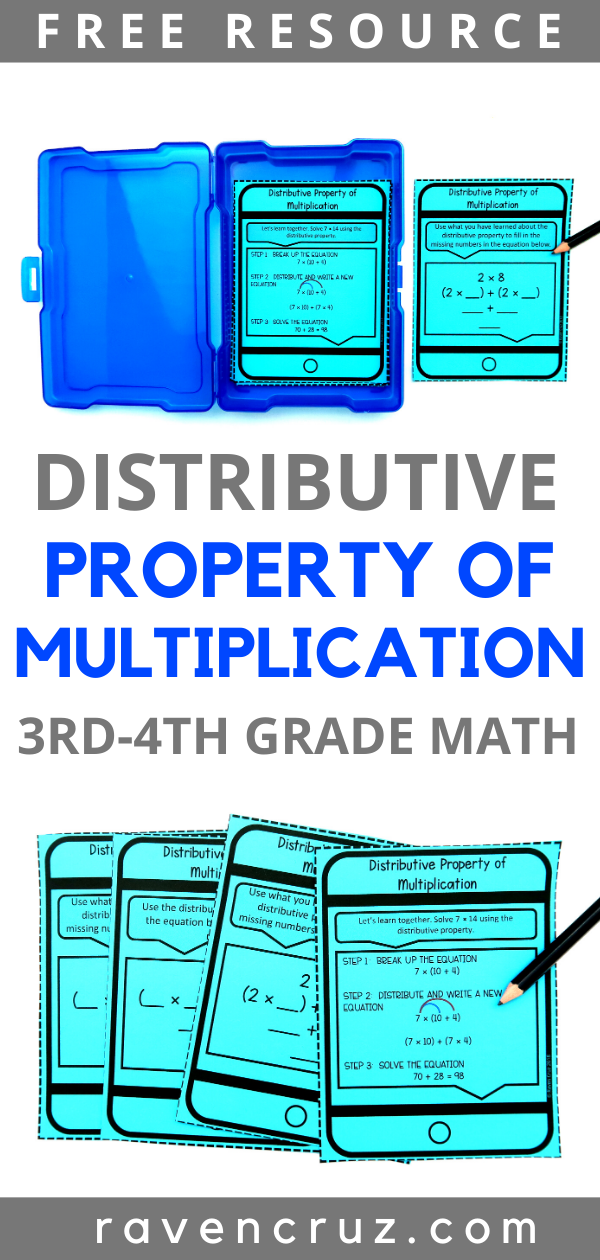Distributive Property of Multiplication Freebie - Raven Cruz

# Distributive Property of Multiplication Freebie

I love getting free quality math resources! Seriously, being a teacher can be expensive! Plus, we love to spend money on our students so when we can save money -- we need to do it. Not only will this distributive property of multiplication freebie for third-grade math save you money, it is truly a helpful freebie.

The properties of multiplication are hard enough to teach, I want to provide something engaging + useful for teachers to use with their curriculum. The task cards work great in math centers, math rotations, etc...

By the way, if you like free resources, sign up for my email list. I love giving my fellow teachers and homeschool parents free resources and tips for their elementary classroom. My email list subscribers get exclusive freebies that aren't available anyone else.## The Distributive Property of Multiplication Task Cards

You can grab this free distributive property resource in my Teachers Pay Teachers store. The free resource is a sneak peek into what comes in the full Properties of Multiplication Task Card product.

## The Distributive Property of Multiplication

The distributive property of multiplication states that when a number is multiplied by the sum of two numbers, the first can be distributed to both of those and multiplied by each separately, then adding the two products together.

First, we "break-up" one of the factors to make it easier for 3rd-graders to multiply. If a student is given the multiplication sentence 8 × 16, it is much easier for the student to "break up" the factor 16

For example:

8 × (10 + 6)

Next, the student will distribute the 8 to the addends and write a new equation.

(8 × 10) + (8 × 6)

Last, solve the equation.

80 + 48 = 128

As you can see, "breaking-up" the factor 16 and distributing the 8 to each addend made the problem much easier to solve. A student does not necessarily have to break 16 up into 10 and 6, but it most cases it is the logical way.